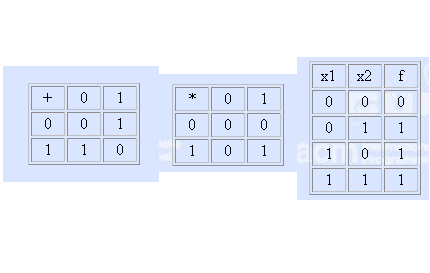# 1979. Binary Polynomials

Each mapping f of the set {0,1}n of n-dimensional binary vectors to {0,1} is called Boolean function of n variables and denoted by f(xn,xn-1,…,x1). For cryptography some properties of the Boolean functions are interesting. Let denote by B(n,k) the set of n-dimensional binary vectors that have k 1’s. The task is for given Boolean function f to find the number of vectors (bn,bn-1,…,b1) from B(n,k) such that f(bn,bn-1,…,b1)=1.

The Boolean function will be given by its (unique) polynomial modulo 2. In these polynomials the operations addition and multiplication modulo 2 are used, defined as shown in the tables of Fig. 1. In the polynomial of a function any product of m variables could participate or not participate. So the general form of the polynomial for n variables is:

a0+a1x1+a2x2+a3x2x1+a4x3+a5x3x1+a6x3x2+a7x3x2x1+. . .+aNxnxn-1…x1

where all coefficients aj, j=0,1,…,N=2^n-1, are 0’s or 1’s and if the coefficient is equal to 0 we will omit the corresponding product and if it is equal to 1 we just will omit the coefficient. For example, the polynomial of the Boolean function disjunction of 2 variables given on Fig. 2 is 0+1.x1+1.x2+1.x2x1=x1+x2+x2x1.### 输入格式

Your program has to be ready to solve more than one test case. The first line of the input will contains only the number T of the test cases. Each of the following T lines will describe one function - first the numbers n and k separated by single space (1 <= n <= 18,0 <= k <= n) and then, separated by one more space a string of 2^n 0’s and 1’s that are the coefficients of the corresponding polynomial, ordered as in the general form of the polynomial given above.

### 输出格式

The output have to contain T lines with a single number each - the number of vectors found by your program.

### 样例

Input
3
2 1 0111
4 2 1000000000000000
5 3 00000000000000000000000000000001

Output
2
6
0


0 人解决，1 人已尝试。

0 份提交通过，共有 1 份提交。

9.9 EMB 奖励。